# Introduction#

A linked list is a collection of sequential elements forming a linear data structure.

In the linked list, each element of the collection is a node storing a value and, depending on the implementation, one or more links to its neighbouring nodes.

This detail makes the linked list a dynamic data structure, which means it can grow or shrink its size.

The doubly linked list is an implementation of a linked list where each node holds a link to the previous node, and to the following node whenever they are present, meaning that a program can traverse a doubly linked list in two directions.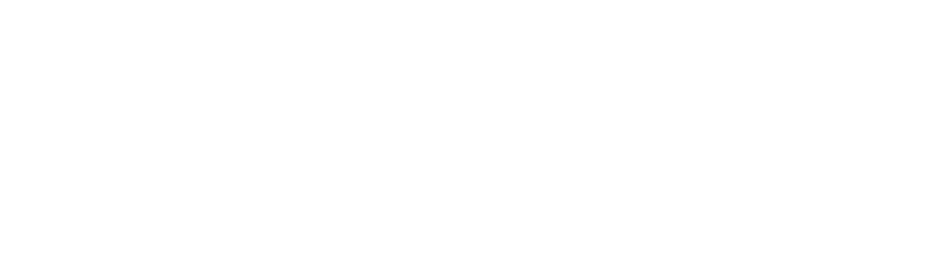# Implementation#

• head: a pointer to the first node of the doubly; when null, it means there are no nodes at all.
• length: to keep track of how many nodes the doubly is storing without traversing the whole sequence.
• mutex: to ensure that the doubly is thread-safe.

Some doubly implementation may also have a tail field, which reduces the cost of insertion/deletion to the end of the linked list; this will not be the case in the following examples.

In Go, an implementation may look like this:

``````type Doubly struct {
mu   sync.Mutex
len  uint
}
``````

Along with the Doubly type, it is mandatory to create a doublyNode type as well, which has three fields:

• value: the value stored in the doubly
• next: a pointer to the next node; when null, it means there are no next nodes
• prev: a pointer to the previous node; when null, it means there are no previous nodes
``````type doublyNode struct {
value interface{}
next  *doublyNode
prev  *doublyNode
}
``````

In this implementation, thanks to an empty interface contract, the doublyNode type allows storing any data type as a value.

Let us go through the behaviour a linked list usually has, and see how those operations work on a doubly linked list.

Adding a new node as part of a doubly, depending on which position it needs to be placed, can be a cheap or an expensive operation.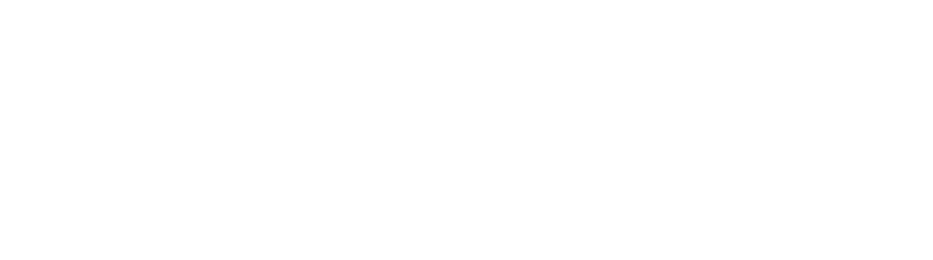A third node needs to be added to the beginning of a doubly holding two nodes.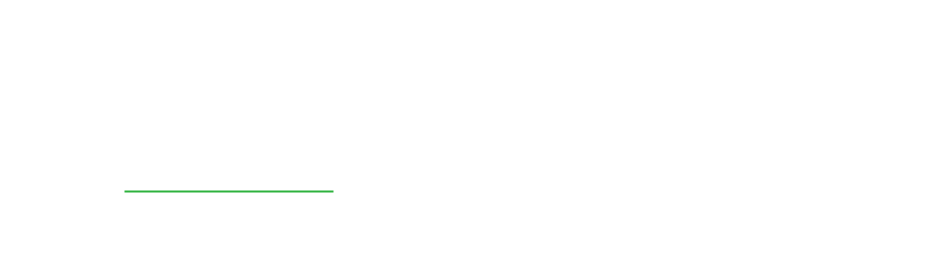Select the head node of the doubly.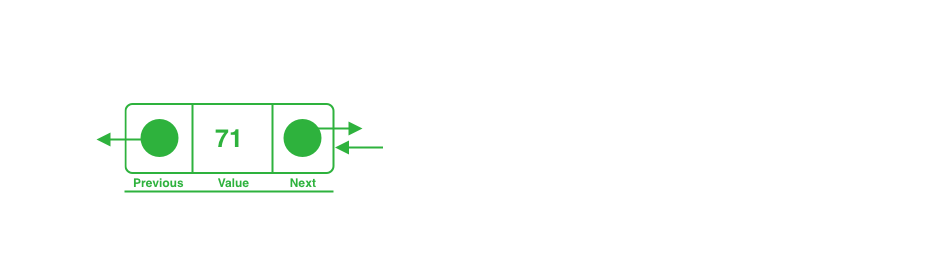Designate the new node as the new head. Link the new head to the former one, and vice versa, to maintain the sequence's integrity.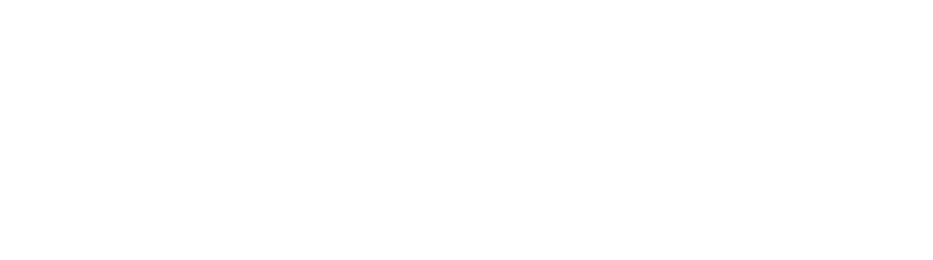Since there is no need to traverse the doubly except accessing the new and old head node, this operation's time complexity is an O(1).

A simple Go method can implement the behavior:

``````func (d *Doubly) AddFront(v interface{}) {
// lock while the operation is ongoing
d.mu.Lock()
defer d.mu.Unlock()

// increase the internal counter of nodes
d.len++

// if there is no head add the first node as such
return
}

// creates a new node and link the next one to the current head
n := &doublyNode{value: v, next: d.head}

// set the head to the early created node and link the previous one
}
``````A third node needs to be added to the end of a doubly holding two nodes.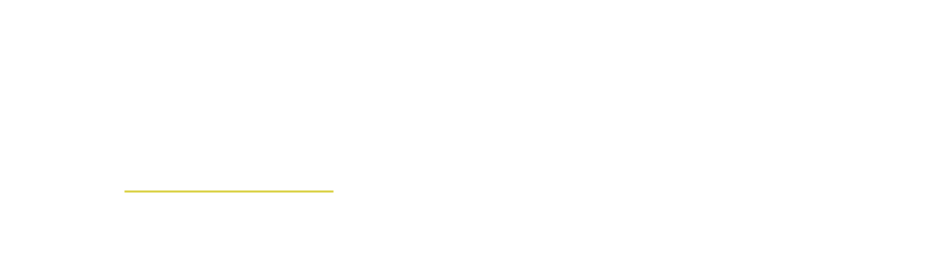Keep moving ahead in the sequence as long as the node is not the last one.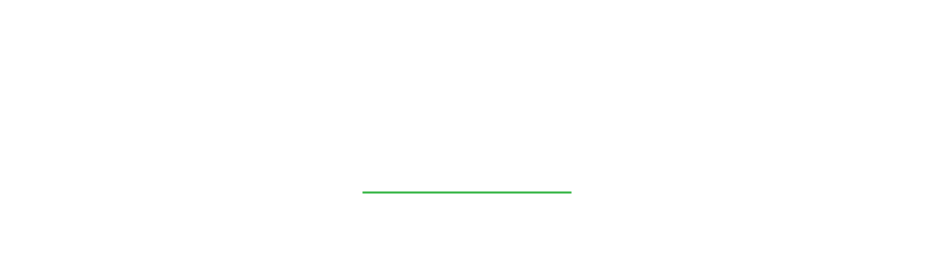Stop traversing the doubly when the last node is found.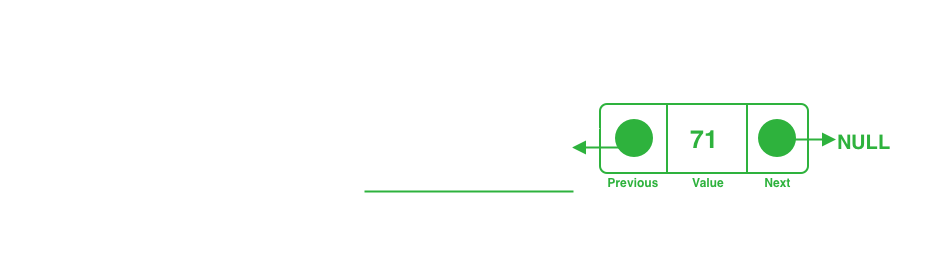Link the last found node to the incoming one and vice versa. As the incoming node will not point to a node, it becomes the last one.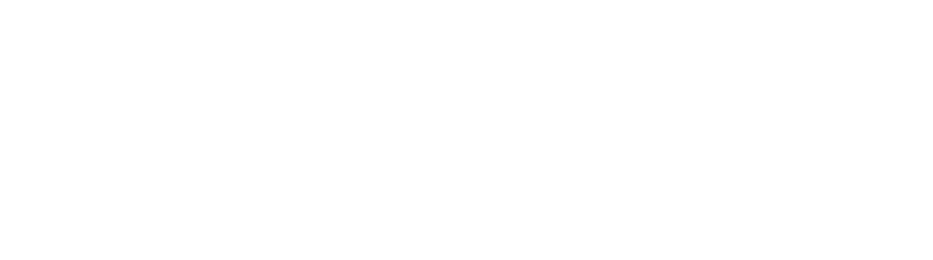Since all the doubly nodes will be traversed, this operation's time complexity is an O(n).

A simple Go method can implement the behavior:

``````func (d *Doubly) AddBack(v interface{}) {
// lock while the operation is ongoing and increase the counter once the function finished
d.mu.Lock()
defer func() {
d.len++
d.mu.Unlock()
}()

// if there is no head add the first node as such
return
}

// as long as `n` is not the last node, proceed moving ahead in the sequence
for i := d.len; i > 1; i-- {
n = n.next
}

// the new node is added as the last in the sequence
n.next = &doublyNode{value: v, prev: n}
}
``````

### Delete#

Deleting a node from a doubly has similar complexity to the add operation.

The position of the node directly influences the time complexity of it.

##### Deleting from front#A node needs to be deleted from the beginning of a doubly holding three nodes.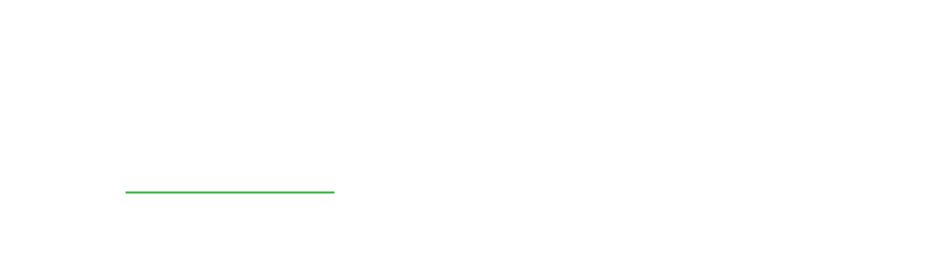Select the head node of the doubly.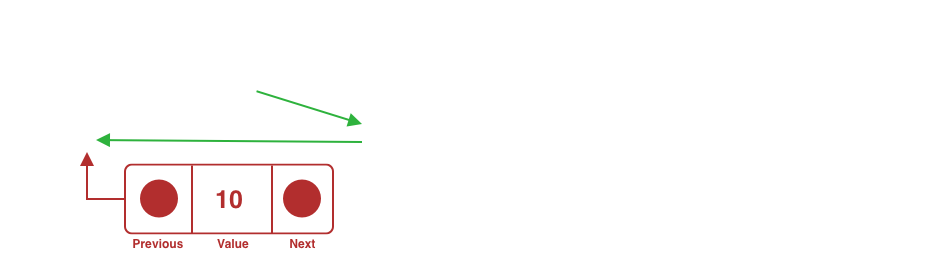Designate the node next to the selected one as the new head and freely drop the former head node.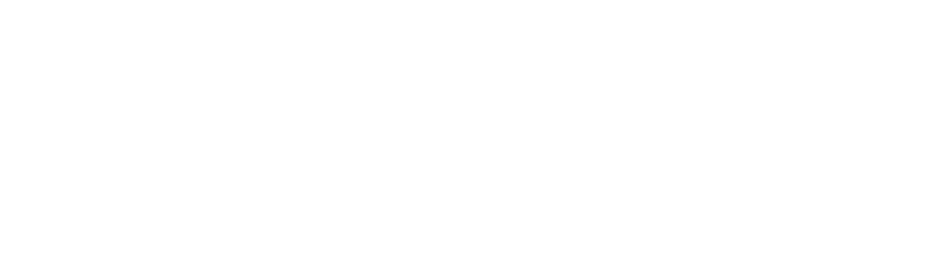Since there is no need to traverse the doubly except accessing the head node, this operation's time complexity is an O(1).

It is possible to implement this behavior by adding a simple method similar to this:

``````func (d *Doubly) DeleteFront() bool {
// lock while the operation is ongoing
d.mu.Lock()
defer d.mu.Unlock()

// if there are no items return false to notify that the operation did not delete any node
return false
}

d.len--

// if the head does not have a next node, delete the head
// and return true to notify a node has been removed
return true
}

// replace the head with its next item
// and return true to notify a node has been removed
return true
}
``````
##### Deleting from the back#A node needs to be deleted from the end of a doubly holding three nodes.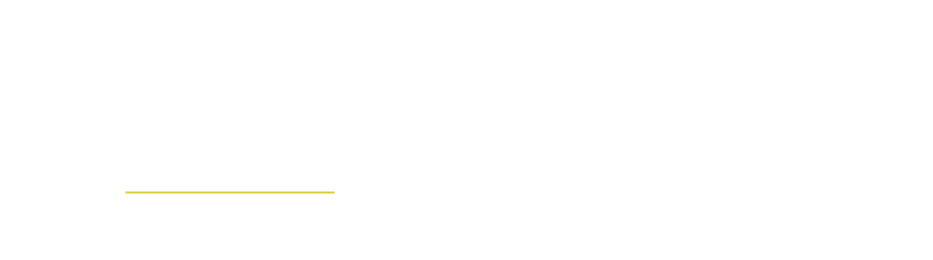Start traversing the doubly from the first node, and keep moving ahead in the sequence as long as the node is not the second-to-last.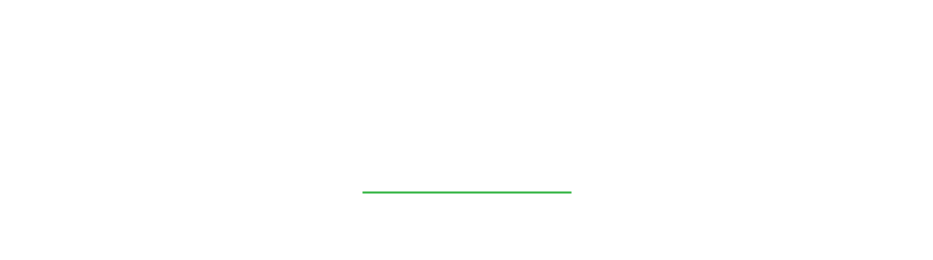Stop traversing the doubly when the second-to-last node is found.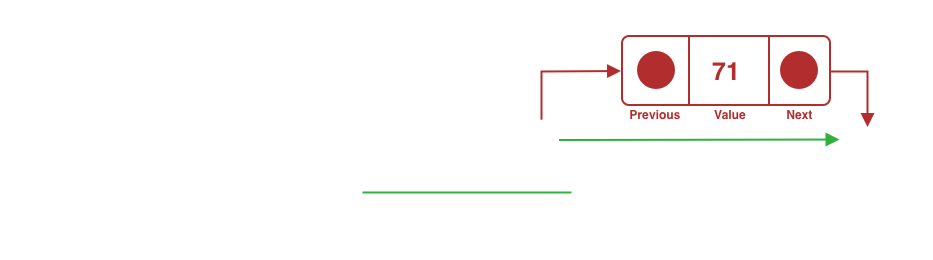The found node needs to link to nothing, excluding the last node from the doubly.Since all the doubly nodes will be traversed, this operation's time complexity is an O(n).

It is possible to implement this behavior by adding a simple method similar to this:

``````func (d *Doubly) DeleteBack() bool {
// lock while the operation is ongoing
d.mu.Lock()
defer d.mu.Unlock()

// if there are no items return false to notify that the operation did not delete any node
if d.len == 0 {
return false
}

// decrease the counter once the function return
defer func() { d.len-- }()

// if there is only the head, delete the node at that position
// and return true to notify a node has been removed
if d.len == 1 {
return true
}

// as long as `n` is not the second last node, proceed moving ahead in the sequence
for i := d.len; i > 1; i-- {
n = n.next
}

// delete the last node
// and return true to notify a node has been removed
n.next = nil
return true
}
``````

### Find#

Finding a value in a doubly has two different outcomes; the first one where an index of the node holding the given value is found, or a second one, where the doubly does not have a node holding the given value.

#### Found#A node index needs to be given back if one of the doubly's nodes has that value.Start traversing the doubly from the first node.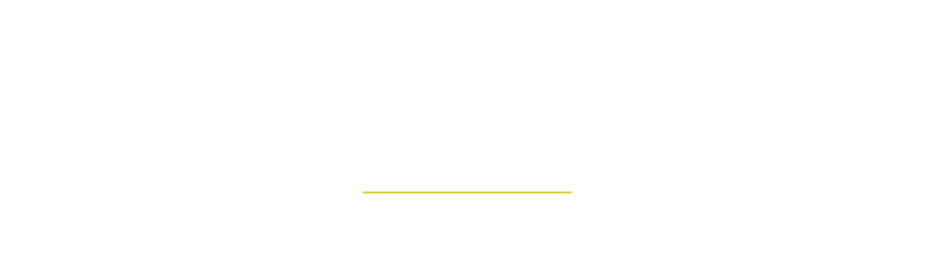Keep moving ahead in the sequence as long as the node does not have the given value.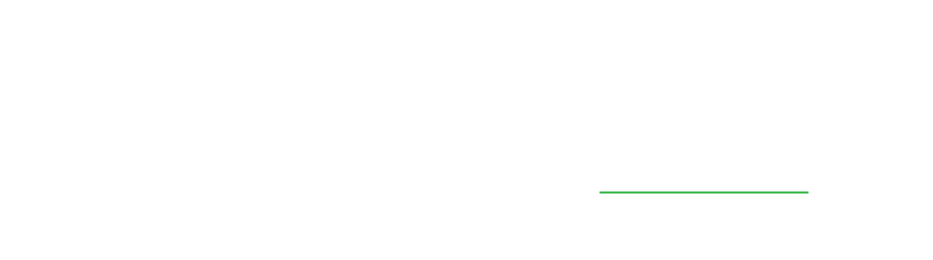Since the last node in the doubly may be the one with the given value, this operation's time complexity is an O(n).A node index needs to be given back if one of the doubly's nodes has that value.Start traversing the doubly from the first node.Keep moving ahead in the sequence as long as the node does not have the given value.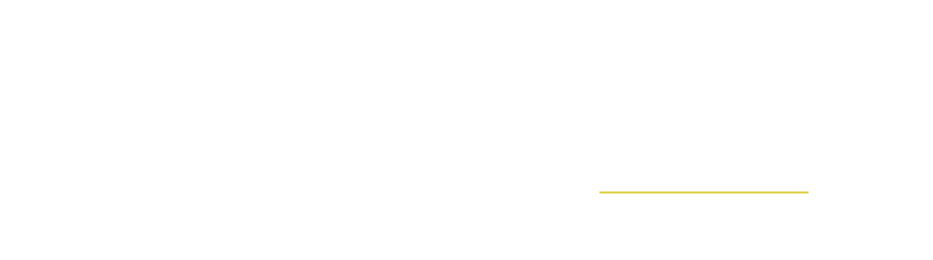When the position is on the last node, it means the value has not been found.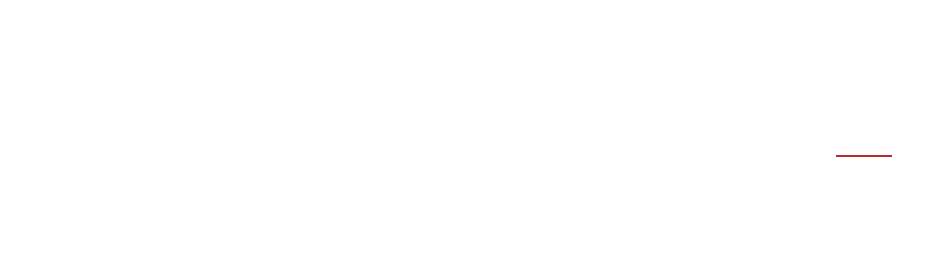Since all the doubly nodes will be traversed, this operation's time complexity is an O(n).

It is possible to implement this behavior by adding a simple method similar to this:

``````func (d *Doubly) Find(v interface{}) (int, bool) {
for i := 0; i < int(d.len); i++ {
switch {
// if `n` is nil, it means we have no nodes in the doubly
// return 0 and false to notify there is no index matching the value
case n == nil:
return 0, false

// if the value is matched then return the index
// and true to mark the success of the operation
case n.value == v:
return i, true

// if none of the previous cases is matched we can move to the next node
default:
n = n.next
}
}

// return 0 and false to notify there is no index matching the value
return 0, false
}
``````

### Iterate#

Iterating a doubly is the last behaviour I am going to share in this article.

The main difference between a singly linked list and a doubly linked list is in the direction during an iteration, this because of the nature of the node that the two use behind the scene.

Since the doubly linked list stores the previous node as well as the next one, it is possible to iterate the nodes in two directions.

#### Iterate forwards#A doubly needs to be traversed from the head to the tail.Start traversing the doubly from the first node.Keep moving ahead as long as there are next nodes.When the position is at the last node, the next node will stop the iteration.Since all the doubly nodes will be traversed, this operation's time complexity is an O(n).

It is possible to implement this behavior by adding a simple method similar to this:

``````func (d *Doubly) IterForwards() func() (value interface{}, found bool) {

// returns a function which will move to the next node
// every time it is called as long as it finds a value
return func() (interface{}, bool) {
if n == nil {
return nil, false
}
defer func() { n = n.next }()
return n.value, true
}
}
``````

#### Iterate backwards#A doubly needs to be traversed from the tail to the head.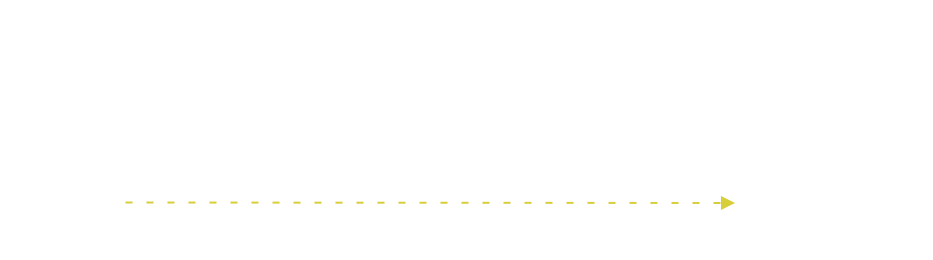Move to the end of the doubly.Start traversing the doubly from the last node.Keep moving backward as long as there are previous nodes.When the position is at the head node, the next node will stop the iteration.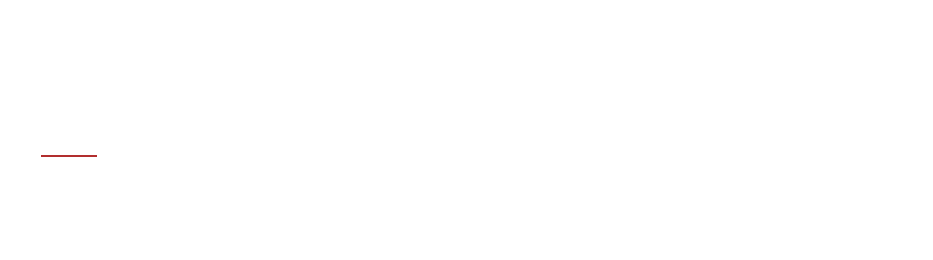Since all the doubly nodes will be traversed, this operation's time complexity is an O(n).

It is possible to implement this behavior by adding a simple method similar to this:

``````func (d *Doubly) IterBackwards() func() (value interface{}, found bool) {
// move to the last node in the doubly
for {
if n == nil || n.next == nil {
break
}
n = n.next
}

// returns a function which will move to the previous node
// every time it is called as long as it finds a value
return func() (interface{}, bool) {
if n == nil {
return nil, false
}
defer func() { n = n.prev }()
return n.value, true
}
}
``````

# Conclusion#

There are many more behaviors that can be part of a doubly linked list, such as adding or deleting a node to a given position, as well as finding a value at a given index and so on.

The doubly linked list is a powerful and robust data structure, which makes perfect sense in use cases where:

• traversing the nodes in two directions is important
• adding or deleting nodes to be efficient and can be done in front
• there is no need for access nodes in the middle of the sequence
• the number of items needs to contain in unknown

To end the article, here is a recap of the time complexity of the operations a doubly linked list can support:

Operation Time complexity